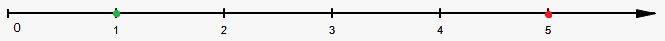# Comparing Whole Numbers

We can compare whole numbers in the following way:

• Greater than. Notation: $>$
• Less than. Notation: $<$
• Equal. Notation: $=$
• Not Equal. Notation: $\ne$
• Greater than or equal. Notation: $\ge$
• Less than or equal. Notation: $\le$

First way to correctly compare whole numbers is use of number line. Remember, that on number line greater number is to the right of smaller number.

For example, since 5 is to the right of 1, then 5 is greater than 1, i.e. ${5}>{1}$ or ${5}\ge{1}$.We just wrote that ${5}>{1}$, but from another side 1 is less than 5 or ${1}<{5}$. This means that we can switch numbers and inequality.

Now, let's do a couple of examples how to determine which sign to apply.

Example 1. Choose correct sign (can be more than one) for 3?4.

Okay, we know that 3 is less than 4 and doesn't equal 4, so correct signs are ${3}<{4}$, ${3}\le{4}$ and ${3}\ne{4}$.

Next example.

Example 2. Choose correct sign (can be more than one) for 5?5.

We need equality signs here! So, ${5}={5}$ or ${5}\ge{5}$ or ${5}\le{5}$.

It is easy to compare small numbers, but what if we can't draw number line for super big number like 2,345,678?

Luckily, there is easy method to determine which number is greater.

How to compare whole numbers?

1. If numbers have different number of digits, add required number of leading zeros until numbers have same number of digits.

2. Start by comparing digits from left to right. If digit in first number is less than digit on same place in second number, then first number is less than second. If digits are equal then examine next to the right digits.

Example 3. Compare 4567 and 12345.

Second number has 5 digits, while first only 4. Thus, we need to add one leading zero to the first number to make it 5-digit.

First number: 04567.

Second number: 12345.

Now compare the leftmost digits 0 and 1.

Since ${0}<{1}$ then ${4567}<{12345}$.

Next example.

Example 4. Compare 345,612 and 2,457.

First number has 6 digits, while second has 4 digits. Thus, we need to add two leading zeros to second number: 2457 becomes 002457.

First number: 345612.

Second number: 002457.

Leftmost digit of first number is 3 and leftmost digit of second number is 0.

Since ${3}>{0}$ then ${345},{612}>{2},{457}$.

Final example.

Example 5. Compare 2345 and 2346.

Numbers have same number of digits, so we don't need to add leading zeros.

First number: 2345.

Second number: 2346.

Compare the leftmost digits 2 and 2: they are equal.

Move to the right: compare 3 and 3. Again they are equal.

Move to the right: compare 4 and 4. Again they are equal.

Move to the right: compare 5 and 6. Since ${5}<{6}$ then ${2345}<{2346}$.

Now, practice a bit.

Exercise 1. Choose correct sign (can be more than one) 15?0.

Answer: ${15}>{0}$, ${15}\ge{0}$, ${15}\ne{0}$.

Next exercise.

Exercise 2. Choose correct sign (can be more than one) 10?19.

Answer: ${10}<{19}$, ${10}\le{19}$, ${10}\ne{19}$.

Next exercise.

Exercise 3. Compare 45673 and 234.

Answer: ${45673}>{234}$. Hint: number of digits is not equal, so add leading zeros to the second number: 234 becomes 00234.

Next exercise.

Exercise 4. Compare 3456 and 3500.

Answer: ${3456}<{3500}$. Hint: ${4}<{5}$.

Last exercise.

Exercise 5. Compare 86743 and 86743.

Answer: Numbers are equal, i.e. ${86743}={86743}$.